# What actually happens when you transfer a model to a custom dataset?

See visually what needs to be altered in the model and how it impacts further training.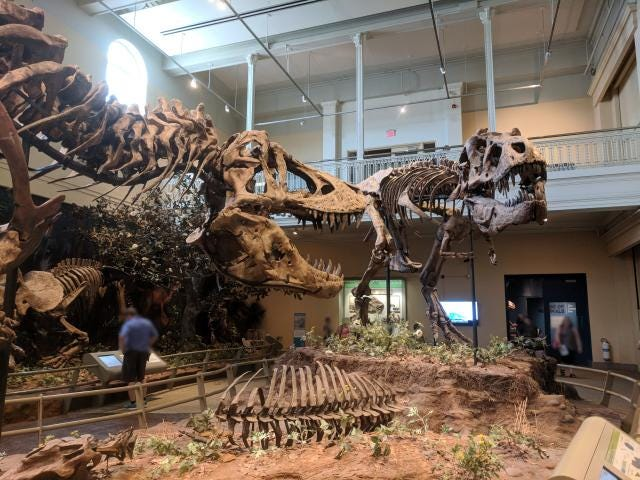It doesn’t have to be scary to understand how neural network models are changed. Photo by author.

One of the great results in Deep Learning came in a method known as transfer learning. That is the situation in which using a network trained for a particular task is used as an initialization for another (close) problem. This is possible, as the first few layers of the network learn generic features, which are useful across multiple domains, with the prediction part of the network tuned for the task at hand. One such example may be using a network capable of recognizing species of trees (task A) to a task of differentiating other vegetation (task B).

In general, this process requires 2 steps:

0. Train/obtain a model for task A.

1. Change the network structure to fit task B.

2. Fine tune the network on task B.

If we want to be a bit more accurate, step 2 would involve freezing the feature extractor and training the head of the network. This stops large magnitude gradients of propagating to the first layers of the network and changing the prior filters. After this stage, it is now possible to unfreeze and train the whole model, thus fine tuning the feature extractors to the exact task at hand.

# Load data

To begin with, let’s load an image and use Efemarai to show it. If you haven’t set it up, register for free and look at the . You should now see a dinosaur skeleton.

`from torchvision import transforms as Tfrom PIL import Imageimport requestsimport efemarai as efurl= "https://data.efemarai.com/samples/dino.jpg"img = Image.open(requests.get(url, stream=True).raw).convert('RGB')ef.inspect(T.ToTensor()(img))`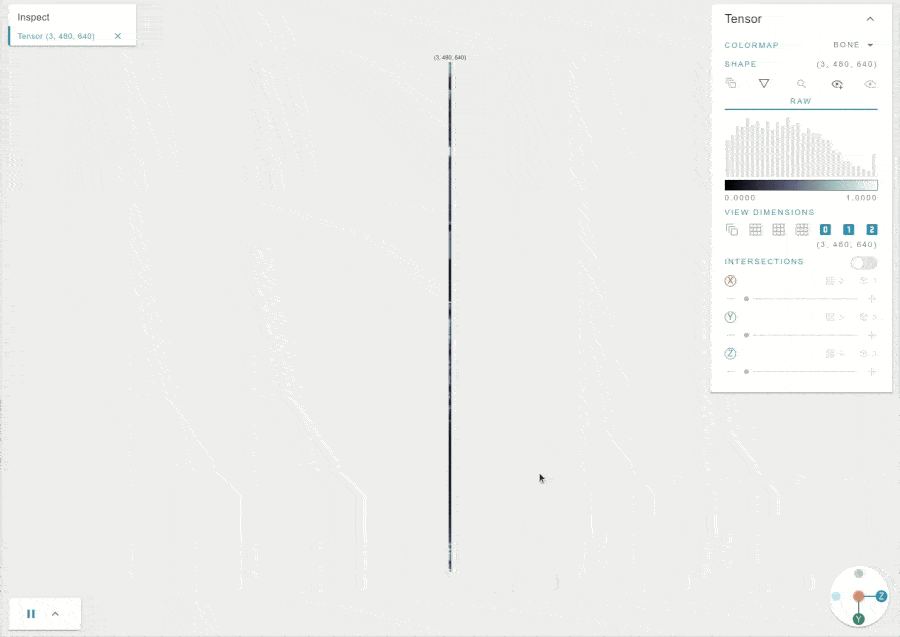Apply ef.inspect() on a tensor or numpy array.

# Step 0 — finding the pre-trained model

For the majority of vision tasks that involve natural scenes, we can use the pre-trained ImageNet model. They are commonly distributed with modern machine learning toolkits and for instance, in PyTorch can be accessed as

`import torchvision.models as modelsresnet18 = models.resnet18(pretrained=True)densenet = models.densenet161(pretrained=True)`

Let’s have a look at a few models. Below we can see the structure of the ResNet model.

`with ef.scan():    output = resnet18(T.ToTensor()(img).unsqueeze())`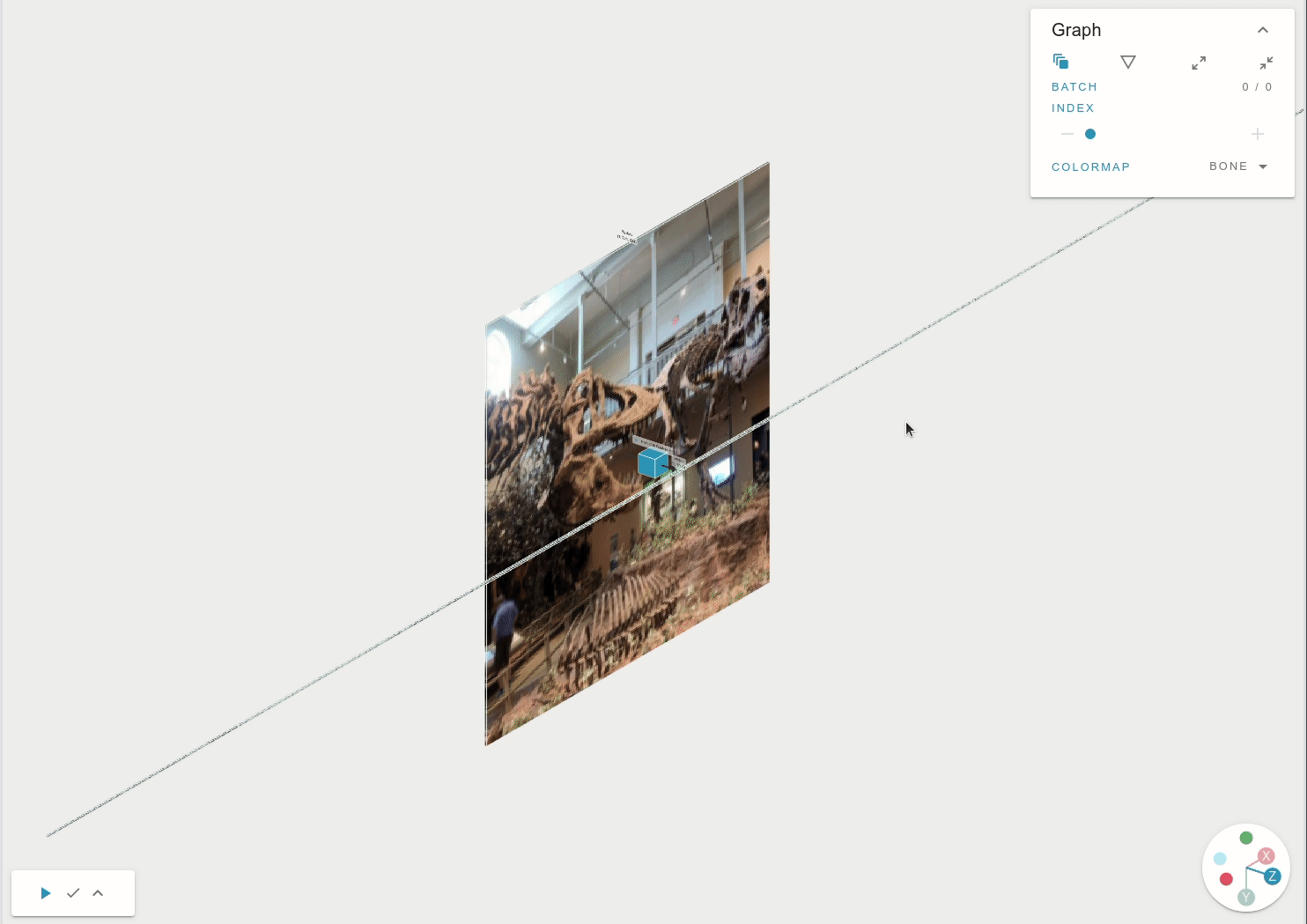ef.scan() tracks the functions that are called and shows the full computational graph of the model. Later on we can see also the loss function, and how to change things.

If we look through the feature maps, we can observe some kernels targeting high frequency (or high fidelity/details) and others are looking at the general structure (low frequency).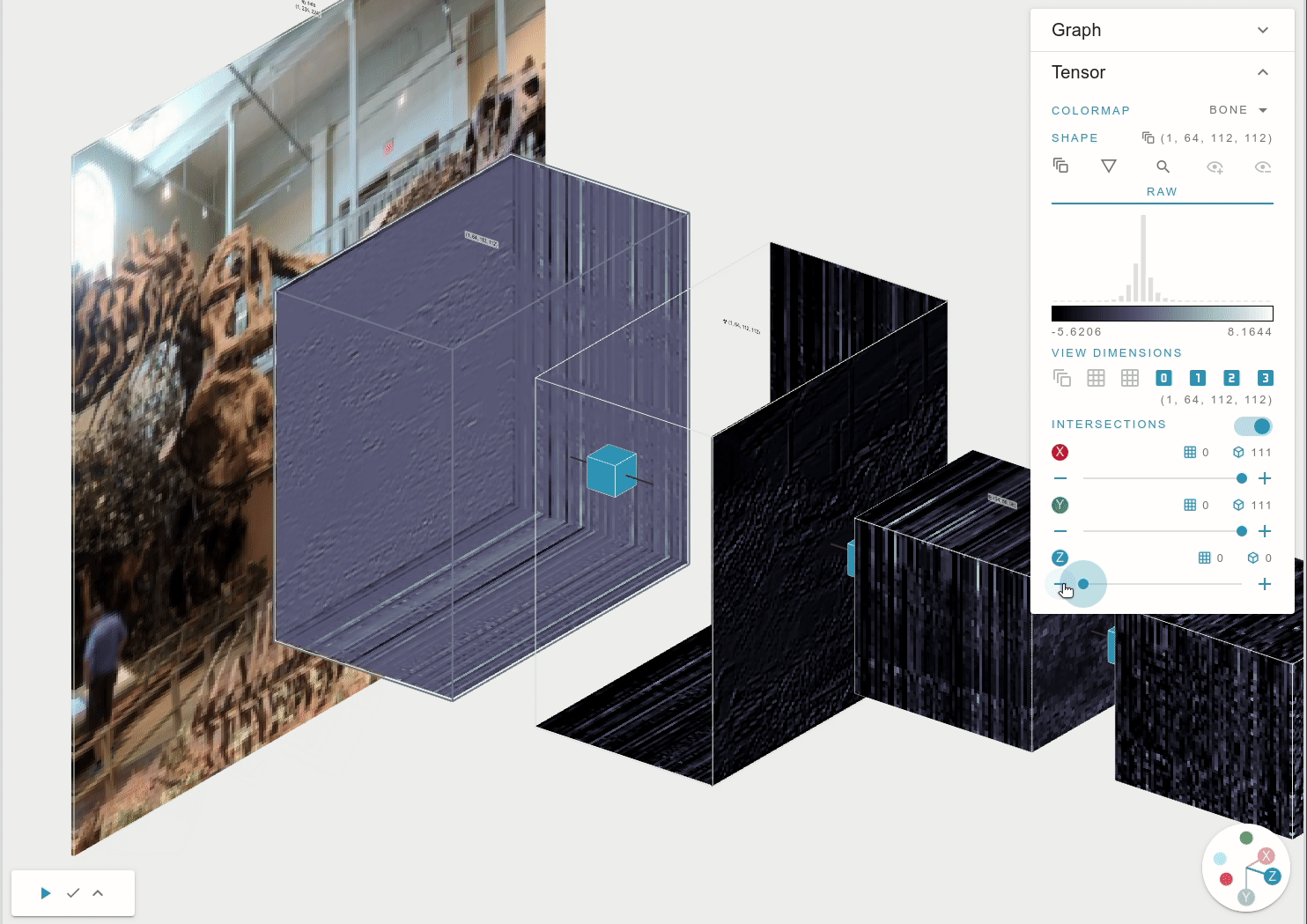You can click on any of the tensors, enable intersections view and scroll through the different layers on the feature maps.

# Step 1

Let’s use the pre-trained ImageNet model and fine-tune it to the CIFAR10 task.

Things to note in the previous image is the output tensor. It has 1x1000 dimensions. This is because the ImageNet task involved classification within a 1000 classes.

If we start training on a custom dataset with a different number of classes. We’ll quickly come across the error of mismatch between the data and the model structure.

What needs to happen is that we cut the network up to the latent variables, and attach a new untrained head targeted at our new problem setup.

Now, let’s navigate down the model and see how the output layer is generated. We can observe that the output is flattened, resulting in a 512 dimensional tensor, which is then connected to a linear layer, generating the output tensor.

When clicking on the block, we can see that the resnet18.fc is actually a torch.nn.functional.linear layer. Expanding further, we can see the large weights associated with the linear layer (512 x 1000 floats).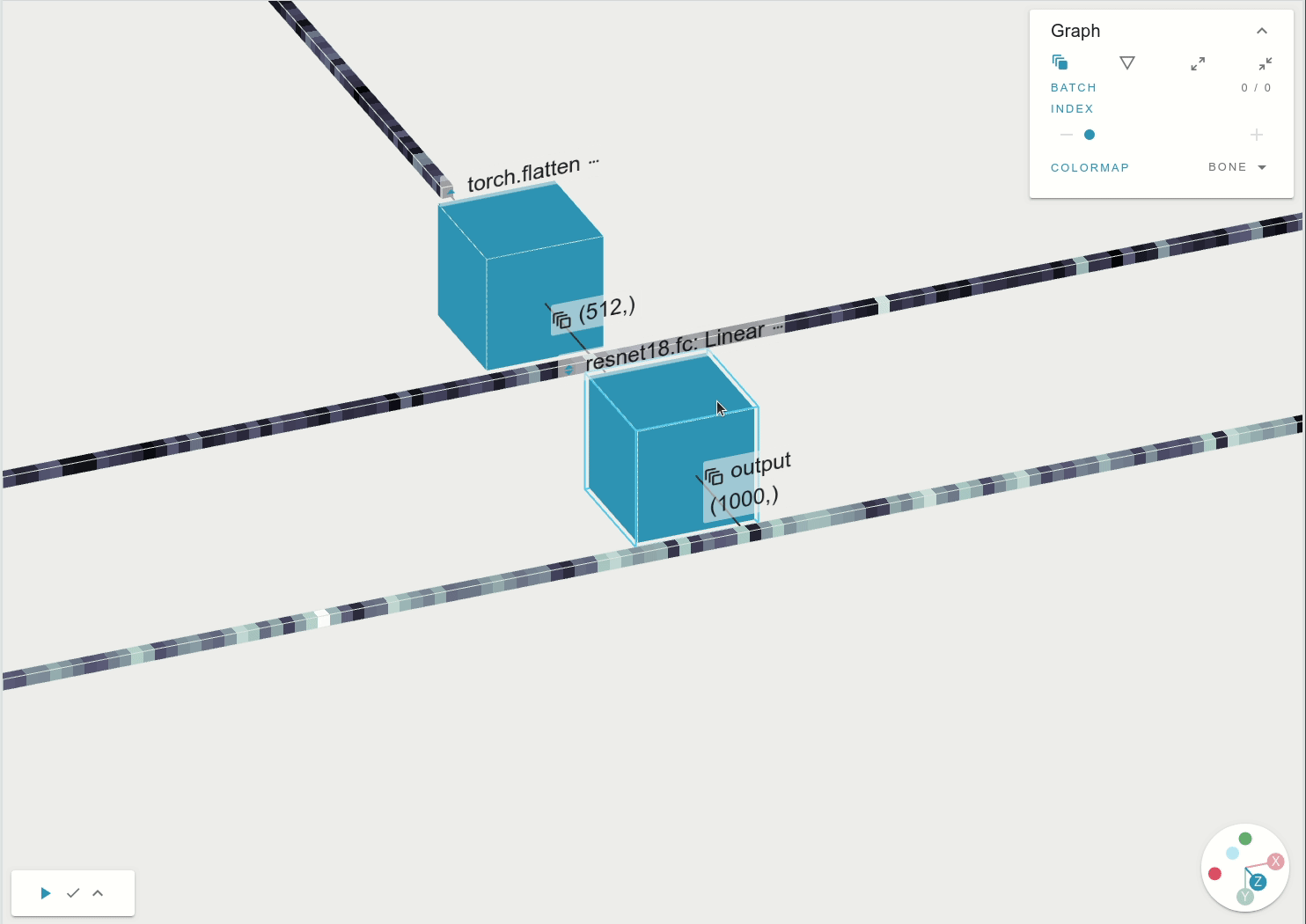Clicking on the function expands internal calls. When expanded fully, we can see the large weight matrix associated with the linear layer. Expansion goes through resnet18.fc:Linear -> torch.nn.functional.linear -> torch.addmm.

And so, to change the computational graph, we can substitute the resnet18.fc variable with a linear layer with the right dimensions. For the task or retraining for CIFAFR10, we need to select the output to have a dimension of 10. And now the weight matrix is much smaller, but it is randomly initialized. Thus not tuned the task at hand.

`import torchnum_ftrs = resnet18.fc.in_featuresresnet18.fc = torch.nn.Linear(num_ftrs, 10)`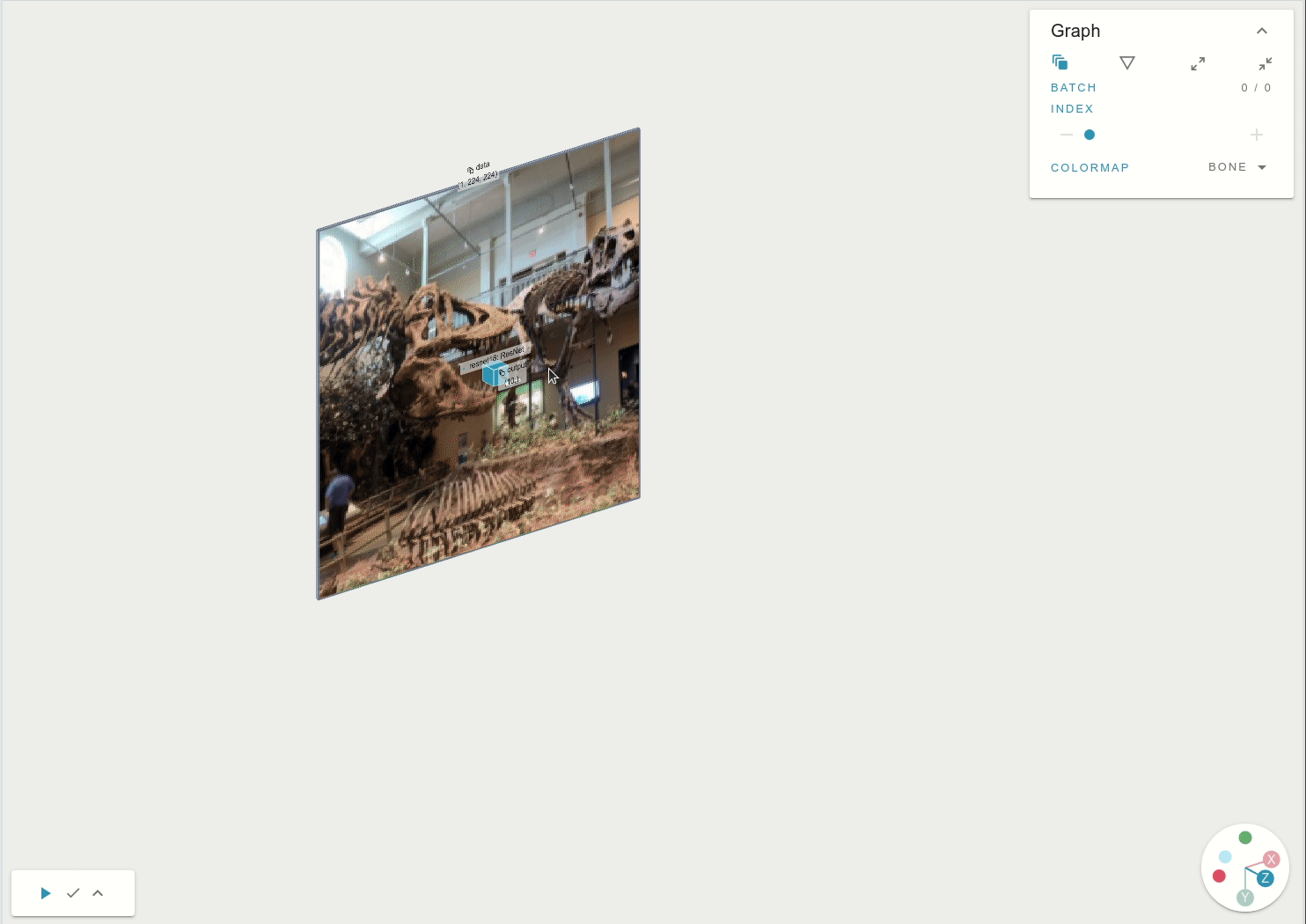The output layer is now much smaller and has a size of 10.

Hurrah! Now we are left with training the model. This is happening on Step 2.

# Step 2 — model training

Here we need to freeze the feature part of the network. Train. Unfreeze, and fine-tine. Let’s introduce the code to load the data, transform it and loop over the examples to calculate the gradients.

`import efemarai as efimport torchimport torchvisionimport torchvision.transforms as transformsfrom torch import nnimport torch.optim as optim## Get data#transform = transforms.Compose(    [transforms.ToTensor(),     transforms.Normalize((0.5, 0.5, 0.5), (0.5, 0.5, 0.5))])trainset = torchvision.datasets.CIFAR10(root='./data', train=True,    download=True, transform=transform)trainloader = torch.utils.data.DataLoader(trainset, batch_size=4,    shuffle=True, num_workers=2)testset = torchvision.datasets.CIFAR10(root='./data', train=False,    download=True, transform=transform)testloader = torch.utils.data.DataLoader(testset, batch_size=4,    shuffle=False, num_workers=2)classes = ('plane', 'car', 'bird', 'cat',           'deer', 'dog', 'frog', 'horse', 'ship', 'truck')## Learning process#criterion = nn.CrossEntropyLoss()optimizer = optim.SGD(resnet18.parameters(),lr=0.0001,momentum=0.9)# Let’s freeze the training in all of the layers, but the FC one we initializedfor name, param in resnet18.named_parameters():  if 'fc' not in name:      param.requires_grad = Falsefor epoch in range(10):  # loop over the dataset     for i, data in enumerate(trainloader, 0):        # get the inputs; data is a list of [inputs, labels]        inputs, labels = data        # zero the parameter gradients        optimizer.zero_grad()        # Let's scan the computation        with ef.scan(wait=False):            # forward + backward + optimize            outputs = resnet18(inputs)            loss = criterion(outputs, labels)            loss.backward()            optimizer.step()print('Finished Training')`

If we switch to the gradients view of the network, we can see that we are generating gradient values only for the last layer, as everything else has `requres_grad=False`. Note that changing the parameters of the optimizer doesn’t mean that other gradients are not calculated, and so we need to explicitly mark those.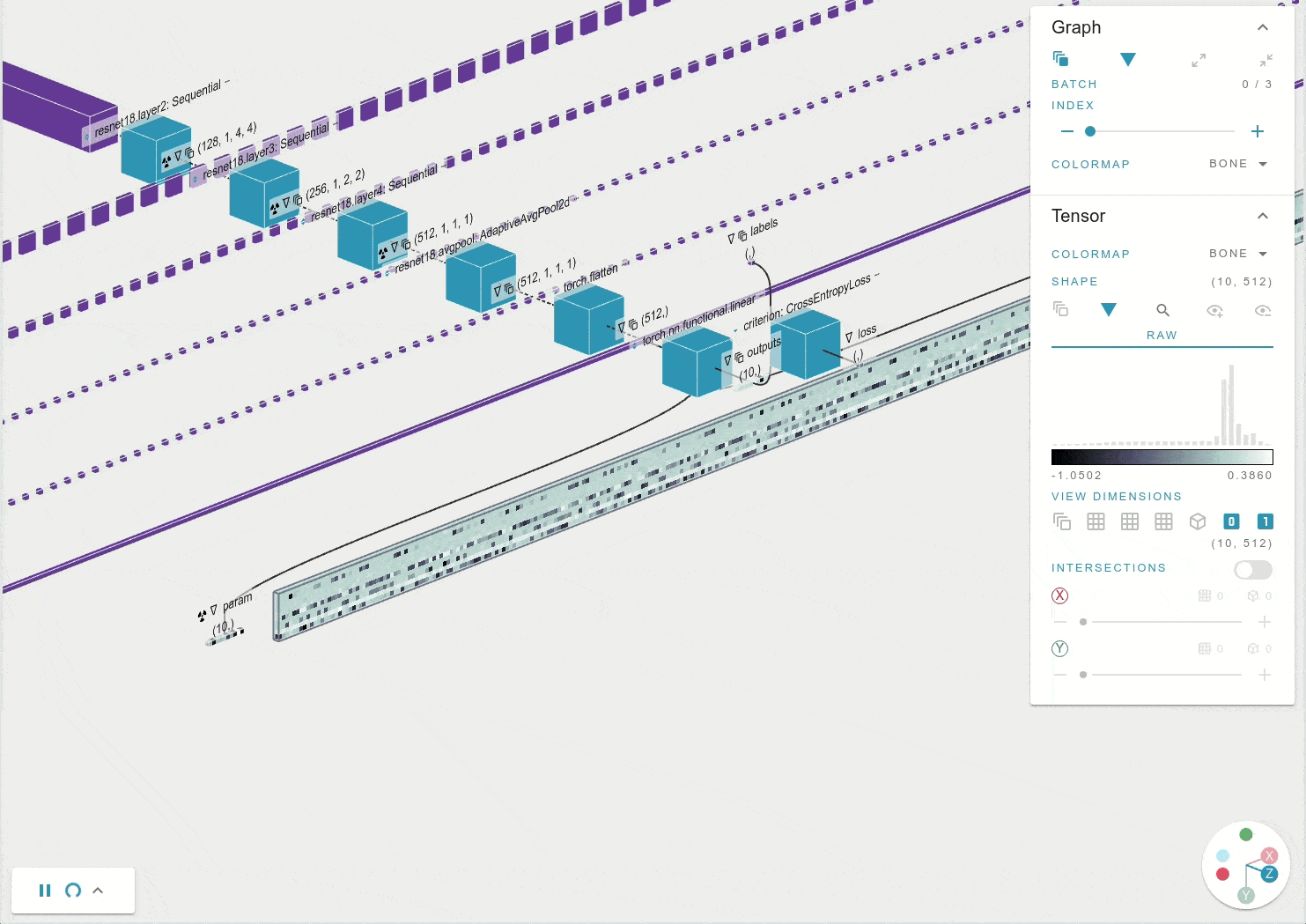You can see that only the gradients on the last layers change. Everything else is not calculated (solid purple color). Speed x4.

Once we are satisfied with the magnitude of the gradients (e.g. after 10–20 epochs), we can set the flag to calculate gradients on all of the parameters. This way, the already tuned final layer would be in a better state than with random initialization. If we scan the graph again, we can see that there are gradients flowing up to the network input. Success!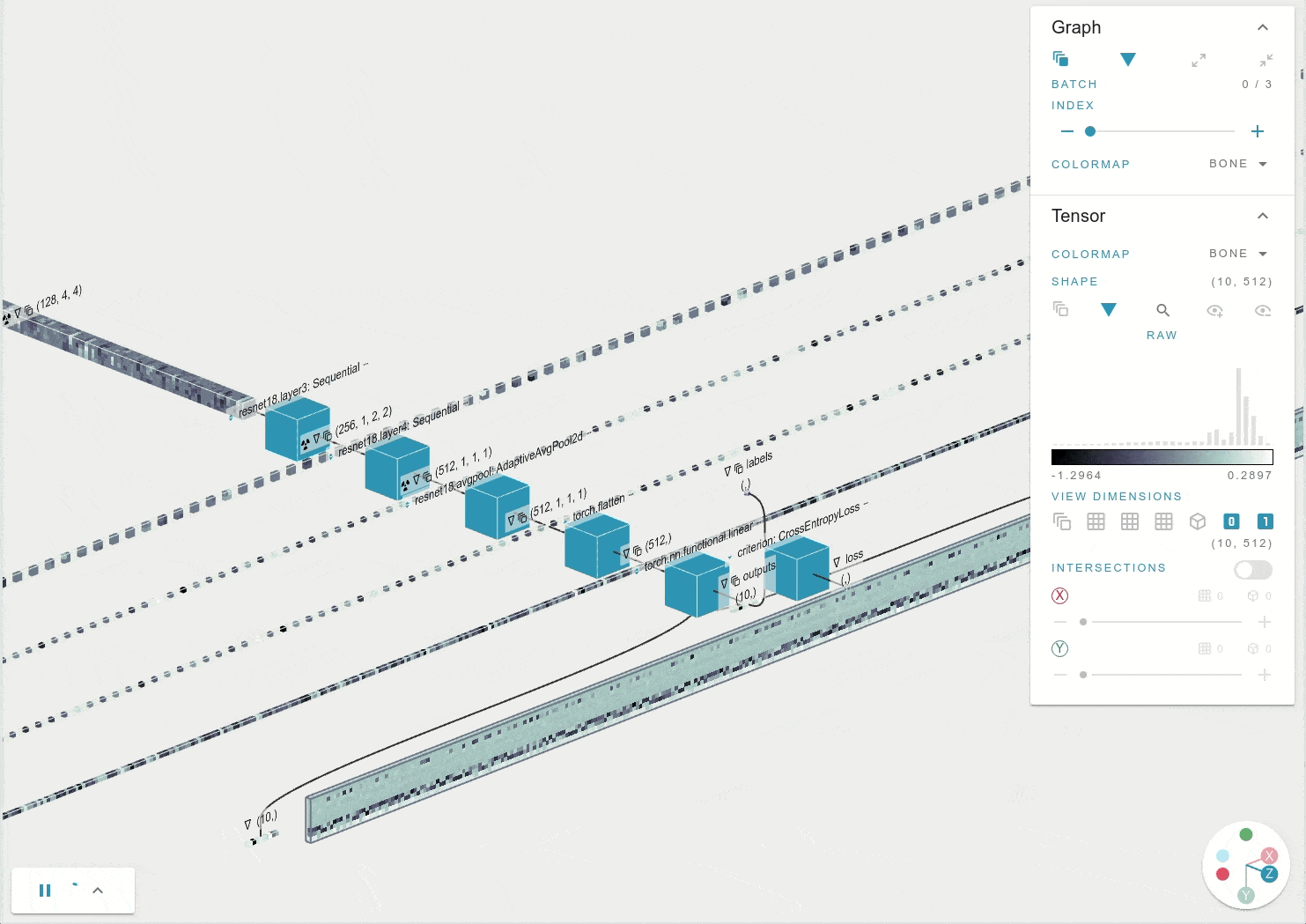Setting requires_grad=True everywhere, allows for the gradient to be computed in all of the weights of the network. Speed x4.

# Common mistakes

A common mistake is providing a single image for inference to the model. The network is expecting to see a batch, and so requires an additional dimension. Have a look at the difference below.

`from torchvision import transforms as Tfrom PIL import Imageimport efemarai as efimg = Image.open("dino.jpg")ef.inspect(T.ToTensor()(img), name='Normal image')ef.inspect(T.ToTensor()(img).unsqueeze(dim=0), name="Unsqueezed")`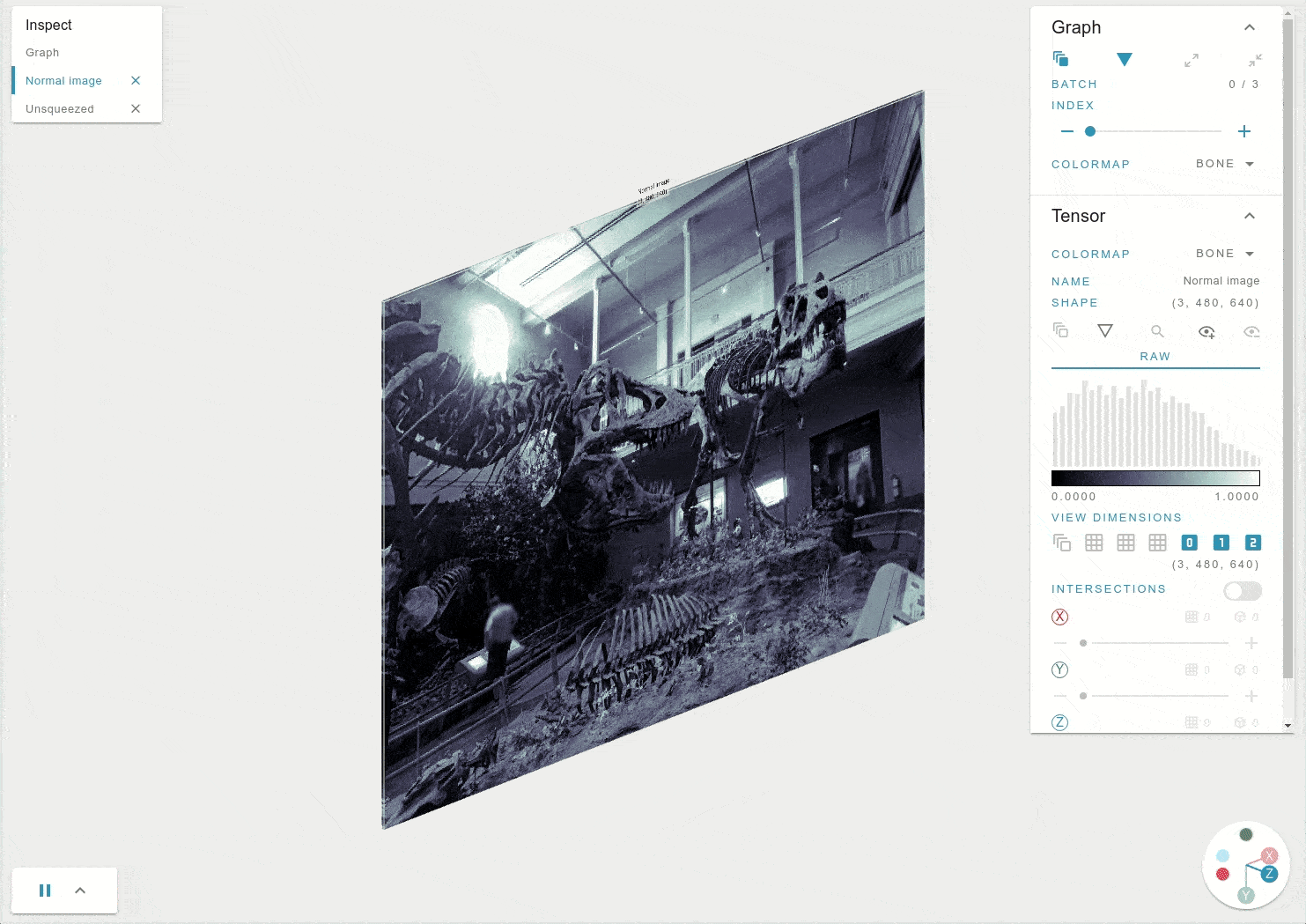The difference between adding a batch size and not.

# Conclusion

What we wanted to show is that understanding the underlying structure of the neural model, helped by interactive debuggers like , allow you to very intuitively change networks, catch when issues happen and not be afraid of going off the beaten path in search for new models.

PhD Machine Learning and Robotics @ University of Edinbrugh

## More from Daniel Angelov

PhD Machine Learning and Robotics @ University of Edinbrugh